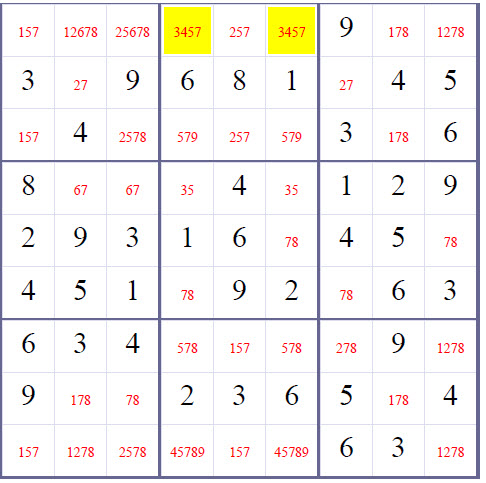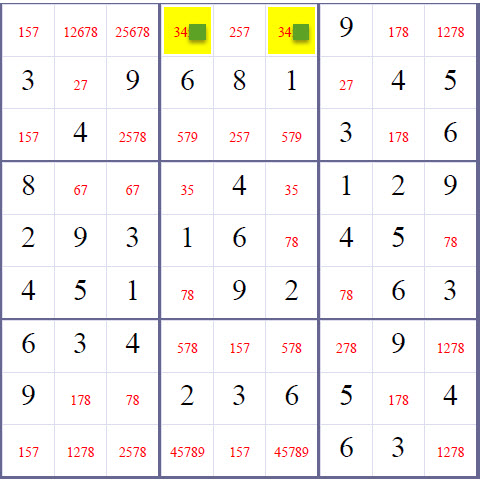Chat

### SubsetPosition2, or Hidden Pair, or Hidden Twins - Advanced Solving Sudoku Technique

SubsetPosition2 by createclassicsudoku.com

a.k.a. Hidden Twins by humage.com

a.k.a. Hidden Pair by hodoku

SubsetPosition2, or Hidden Twins, or Hidden Pair:

If you can find two blank/empty within a row(or column, or box) such as that two candidates appear nowhere outside those cells in that row (or column, or box), those two candidates must be placed in the two cells. All other candidates can therefore be eliminated.

Candidate Numbers (or Candidate Values) of an blank/empty square is a list of possible values or candidates for this blank/empty cell.

Below is an example of using SubsetPosition2(a.k.a. Hidden Pair, or Hidden Twins) to refine the candidate values of R1C4 and R1C6 (the highlighted squares). Blank/Empty Squares are marked with candidate values in red.

Hard Sudoku on 03/16/2018For the first row, only R1C4 and R1C6 contains candidate values 3 and 4. Either number 3 in R1C4 and number 4 in R1C6, or number 4 in R1C4 and number 3 in R1C6. So we can conclude that numbers 3 and 4 must be in R1C4 and R1C6. And we can safely remove other numbers (besides 3 and 4) from R1C4 and R1C6's candidate values.For convenience, in our Sudoku solver, we call

Subset2Row:

an untaken number in a row only show up in one empty square as its candidate values, then this empty square must take the number.

Subset2Col:

an untaken number in a column only show up in one empty square as its candidate values, then this empty square must take the number.

Subset2Box:

an untaken number in a 3×3 box only show up in one empty square as its candidate values, then this empty square must take the number.

### How to find them?

• Use basic methods to solve the Sudoku until no further blank/empty square can be inferred to be a number using basic methods (Sole Candidate and Hidden Single).
• Write down the candidate values for every blank/empty square.
• Check whether there exist two blank/empty squares which are the only squares containing two untaken numbers in a row(or column, or box).
• When there are two blank/empty squares which are the only squares containing two untaken numbers in a row(or column, or box), remove all other candidate values from these two squares.

##### Solving Sudoku Methods Explained

This article answers questions: What are commonly used solving sudoku methods? What solving Sudoku methods do we use? In what sequences?

##### Basic Solving Sudoku Method: One Choice

a.k.a Sole Candidate, Only Candidate, or Naked Single

##### Basic Solving Sudoku Method: Elimination

a.k.a Hidden Single, Loner

##### Advanced Solving Sudoku Method: Subset2

a.k.a Pair, Naked Pair, or Naked Twins

##### Advanced Solving Sudoku Method: SubsetPosition2

a.k.a Hidden Pair or Hidden Twins

Last updated 3 mins ago

##### Advanced Solving Sudoku Method: Interaction

a.k.a Box/Row Claim or Intersection , or Locked Candidates

##### Solving Sudoku Methods Explained

This article answers questions: What are commonly used solving sudoku methods? What solving Sudoku methods do we use? In what sequences?